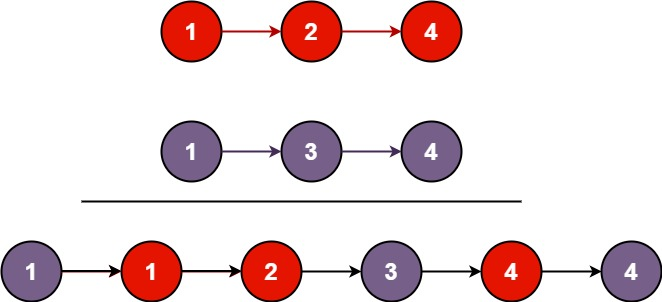# 21. Merge Two Sorted Lists / Easy

Merge two sorted linked lists and return it as a sorted list. The list should be made by splicing together the nodes of the first two lists.

## Example 1:Input: l1 = [1,2,4], l2 = [1,3,4]
Output: [1,1,2,3,4,4]

## Example 2:

Input: l1 = [], l2 = []
Output: []

## Example 3:

Input: l1 = [], l2 = 
Output: 

## Comstraints

• The number of nodes in both lists is in the range [0, 50].
• -100 <= Node.val <= 100
• Both l1 and l2 are sorted in non-decreasing order.

# Solution 1: Vector

## 效能

### Complexity

• Time Complexity: O((N+M)log(N+M))
• Space Complexity: O(N+M)

### LeetCode Result

• Runtime: 9 ms
• Memory Usage: 15.1 MB

# Solution 2: LL Operation

## 效能

### Complexity

• Time Complexity: O(N+M)
• Space Complexity: O(1)

### LeetCode Result

• Runtime: 4 ms
• Memory Usage: 14.8 MB

# Solution 3: Recursion

## 效能

### Complexity

• Time Complexity: O(N+M)
• Space Complexity: O(N+M)

### LeetCode Result

• Runtime: 4 ms
• Memory Usage: 14.8 MB